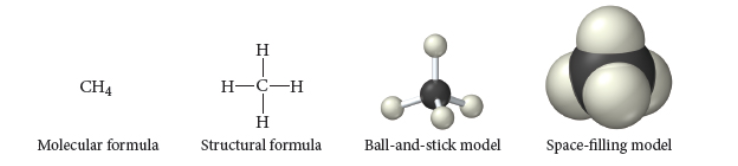# Problem: sp in the space-filling molecular models shown below represent? If you were to superimpose a nucleus on one of these spheres, how big would draw it?(a) Each sphere represents the nucleus of an atom. The nucleus is the same size as the sphere.(b) Each sphere represents the hard outer shell of an atom. The nucleus would be too small to see on the same scale.(c) Each sphere represents the electron cloud of the atom. The nucleus would be too small to see on the same scale.

###### FREE Expert Solution

sphere → electron cloud of the atom

nucleus → too small to see on the same scale

87% (184 ratings)###### Problem Details

sp in the space-filling molecular models shown below represent? If you were to superimpose a nucleus on one of these spheres, how big would draw it?(a) Each sphere represents the nucleus of an atom. The nucleus is the same size as the sphere.

(b) Each sphere represents the hard outer shell of an atom. The nucleus would be too small to see on the same scale.

(c) Each sphere represents the electron cloud of the atom. The nucleus would be too small to see on the same scale.

Frequently Asked Questions

What scientific concept do you need to know in order to solve this problem?

Our tutors have indicated that to solve this problem you will need to apply the Molecular Models concept. If you need more Molecular Models practice, you can also practice Molecular Models practice problems.